# Initial conditions

(diff) ← Older revision | Latest revision (diff) | Newer revision → (diff)

Conditions imposed in formulating the Cauchy problem for differential equations. For an ordinary differential equation in the form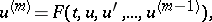(1)

the initial conditions prescribe the values of the derivatives (Cauchy data):(2)

where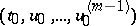is an arbitrary fixed point of the domain of definition of the function; this point is known as the initial point of the required solution. The Cauchy problem (1), (2) is often called an initial value problem.

For a partial differential equation, written in normal form with respect to a distinguished variable,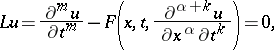the initial conditions consist in prescribing the values of the derivatives (Cauchy data)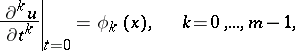of the required solution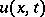on the hyperplane(the support of the initial conditions).